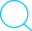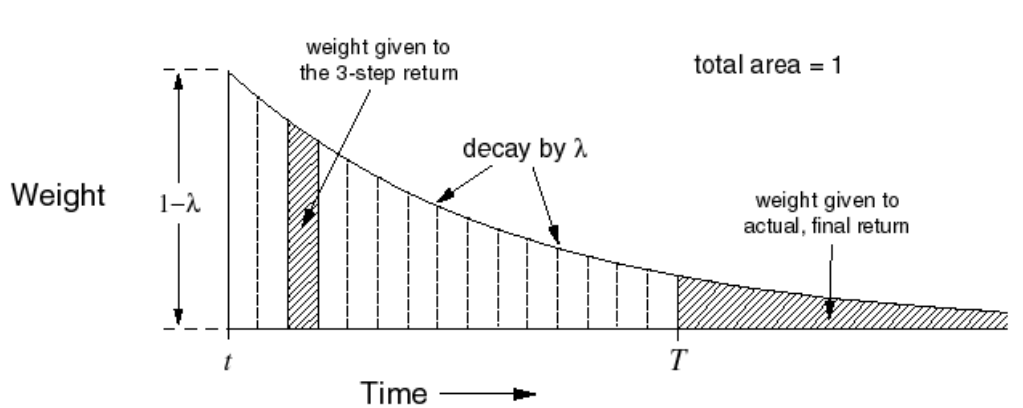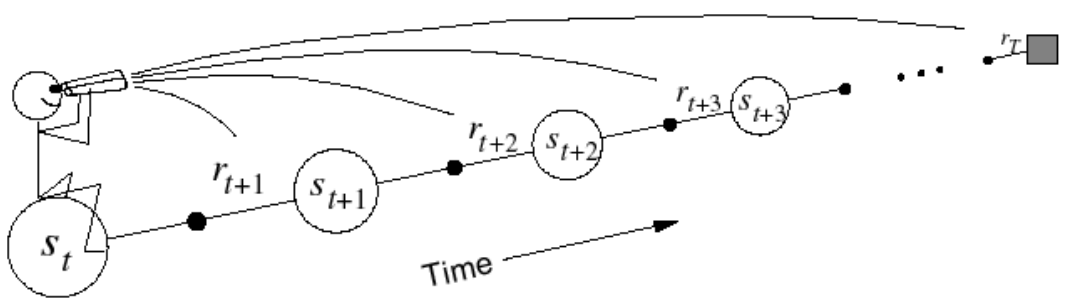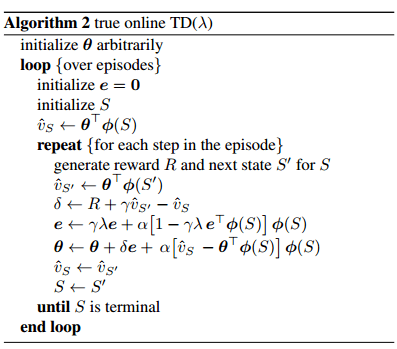16 Mar 2017

# Model-free prediction algorithms for deep reinforcement learning --Similarities and differences (WIP)

Conventions:

• Sets are represented by $\mathcal{A, S},…$ (caligraphy font)
• Vectors are represented by $\mathbf{w, \theta,…}$ (bold font)
• Functions are represented by $Q,\hat{Q}, V, \hat V,…$ (capital letters)
• Random variables are represened by $s,a,r…$ (lower case letters)

Note: This post is for comparing the differences and understanding the similarities of various model-free prediction algorithms for (deep) reinforcement learning (especially with function approximations). Red colored fonts indicates the comparable differences (if applicable) from the preceding equation/algorithm. Some of the details may be left out for brievity. Please refer to Sutton & Barto, 2017 and the cited papers for completeness.

## N-step return with value function approximation

\begin{align}g_{t,\mathbf{w_t}}^{(n)}=r_{t+1}+ \gamma * r_{t+2} + \gamma ^2 * r_{t+3} + ...\gamma^{n-1}*r_{t+n}+\gamma^n * \hat V(s_{t+n},\mathbf w_t)\end{align}

equivalently, \begin{align}g_{t,\mathbf w_t}^{(n)}= \sum_{i=1}^n \gamma^{i-1} * r_{t+i} + \gamma^{n} * \hat V(s_{t+n},\mathbf w_t)\end{align}

## $\mathbf{\lambda-return}$ with value function approximation

\begin{align}g_{t,\mathbf{w_t}}^{\color{red}{(\lambda)}}= (1-\lambda) \sum_{n=1}^{\infty}\lambda^{n-1}g_{t,\mathbf{w_t}}^{(n)}\end{align}

The $\lambda-return$, $g_{t,\mathbf{w_t}}^{\lambda}$ combines all n-step (from the current time step) returns using $\lambda^{n-1}$ as the weights for each of the n-step returns. $(1-\lambda)$ is the normalizing term so that, $(1-\lambda) \sum_{n=1}^{\infty}\lambda^{n-1}=1$. Visually, it makes intuitive sense:## $\lambda-return$ with value function approximation for $\color{red}{episodic}$ MDPs

Let T be the time step at which a terminal state is reached which marks the end of an episode. Therefore, the number of steps that can be taken from the current time step t until the end of the episode is $T-t$ .

\begin{align}g_{t,\mathbf{w_t}}^{(\lambda)}= (1-\lambda) \sum_{n=1}^{\color{red}{T-t}}\lambda^{n-1}g_{t,\mathbf{w_t}}^{(n)}}\end{align

(Question: Is the normalizing factor of $(1-\lambda)$ still valid? If n goes till $\infty$, summation of $\lambda^{n-1}$ approaches $\frac{1}{1-r}$. For the summation still $T-t$, the term comes to $\frac{1-\lambda^{T-t-1}}{1-\lambda}$. Is the term $1-\lambda$ still the normalizing factor? )

We can pull out the last term from this summation and write it as:

\begin{align}g_{t,\mathbf{w_t}}^{(\lambda)}= \underbrace{(1-\lambda) \sum_{n=1}^{\color{red}{T-t-1}}\lambda^{n-1}g_{t,\mathbf{w_t}}^{(n)}}_{\text{For steps until the end of episode}} + \underbrace{\color{red}{\lambda^{T-t-1}g_{t,\mathbf{w_t}}^{(T-t)}}}_{\text{For step(s) after end of episode}}\end{align}

By the definition of an episodic MDP, after the end of an episode (i.e after a terminal state is reached), there is nothing for an agnet to do. By observation, the last n-step return, ${\color{green}{\lambda^{T-t-1}g_{t,\mathbf{w_t}}^{T-t}}}$ is $\color{green}{equal}$ to the full return, $\color{green}{g_{t,\mathbf{w_t}}}$ of the episode.

\begin{align}g_{t,\mathbf{w_t}}^{(T-t)}=\sum_{i=1}^{T-t}\gamma^{i-1}r_{t+i}=g_{t,\mathbf{w_t}}\end{align}\nonumber

## Truncated $\lambda-return$

Off-line^1 TD($\lambda$) is equivalent to the (off-line) $\lambda-return$ algorithm Sutton & Barto; 1998,Sutton; 1988. However, online^2 TD($\lambda$) is only approximately equal to the online $\lambda-return$ algorithm. Seijen & Sutton; 2014 proposed the use of truncated $\lambda-return$ that truncates the $\lambda-return$ at a specific timestep $t’$ to pave way for the strict-online forward view algorithm.

\begin{align}g_{t,\mathbf w_t}^{\color{red}{\lambda|t'}}= (1-\lambda) \sum_{n=1}^{\color{red}{t'-t-1}} \lambda^{n-1}g_{t,\mathbf {w_{\color{red}{t+n-1}}}}^{(n)}+\lambda^{\color{red}{t'-t-1}} g_{t,\mathbf w_{\color{red}{t'-t}}}^{\color{red}{t'-t}}\end{align}

Updates are accumulated after every step within an episode but applied in batch at the end of the episode.

##### Online update:

Updates are applied online at each time step within an episode

## Forward view

For each visited state, look forward in time to all the subsequent rewards and the sates visited to determine its update.# Temporal Difference Learning with function approximation for $\color{blue}{on-policy}$ model-free prediction

At time step $t+1$, the error at timestep $t$ is used to adjust the estimate of $V(s_t,\mathbf{w_t})$ through the following weight (of the State-value function approximator) update:

## Online forward view (?) TD(0)

$\Delta \mathbf{w_{t+1}}= \alpha * [ r_{t+1} + \gamma * \hat V(s_{t+1},\mathbf{w_t}) - \hat V(s_t,\mathbf{w_t})] \nabla_w \hat V(s_t,\mathbf{w_t})$ \begin{align}\Delta {\mathbf {w_{t+1}}} = \alpha * \delta_t^{(0)} \nabla_w\hat V(s_t,\mathbf w_t )\end{align}

TD(0) updates the weights using one-step return, $r_{t+1} + \gamma * \hat V(s_{t+1},\mathbf w_t)$. This update depends on the immediate reward, $r_{t}$ and the current (at time step $t$) approximation of the value of the next state, $\hat V(s_t,\mathbf w_t)$.

## Strict online-forward view

$\Delta\mathbf{w_{t+1,k}}=\alpha_{k-1}[g_{k-1}^{\lambda|t}-\hat V(s_{k-1},\mathbf{w_{t,k-1}})] \nabla_{\mathbf{w_{t,k-1}}}\hat V(s_{k-1},\mathbf{w_{t,k-1}})$

## The vanilla/conventional Backward view TD($\lambda$)

$\Delta \mathbf{w_{t+1}}=\alpha * [ r_{t+1} + \gamma * \hat V(s_{t+1},\mathbf{w_t})-\hat V(s_t, \mathbf w_t)]*\mathbf e_{t,\mathbf w_t}(s_t)$

or \begin{align}\Delta \mathbf{w_{t+1}}=\alpha * \delta_t*\mathbf e_{t,\mathbf w_t}(s_t)\end{align}

where,

$\mathbf e_{t,\mathbf{w_t}} (s_t)= \gamma \lambda e_{t-1,\mathbf w_t}(s_t) +\nabla_{\mathbf w_t}\hat V(s_t, \mathbf w_t)$

and \begin{align}\delta_t= r_{t+1} + \gamma * \hat V(s_{t+1},\mathbf{w_t})-\hat V(s_t, \mathbf w_t)\end{align}

## True online TD($\lambda$)

True online TD($\lambda$) forms the backward view of the truncated $\lambda-return$ algorithm. Like the vanilla TD($\lambda$), it updates the weights proportional to a decaying eligibility trace.

\begin{align}\Delta \mathbf{w_{t+1}}=\alpha * [ r_{t+1} + \gamma * \hat V(s_{t+1},\mathbf{w_t})-\hat V(s_t, \mathbf w_t)]*\mathbf e_{t,\mathbf w_t}(s_t)\color{red}{+\alpha_t[\hat V(s_t,\mathbf{w_{t-1}})-\hat V(s_t,\mathbf{w_{t}})]\nabla_{\mathbf w_t}\hat V(s_t, \mathbf w_t)}\end{align}

or \begin{align}\Delta \mathbf{w_{t+1}}=\alpha * \delta_t*\mathbf e_{t,\mathbf w_t}(s_t)\color{red}{+\alpha_t[\hat V(s_t,\mathbf{w_{t-1}})-\hat V(s_t,\mathbf{w_{t}})]\nabla_{\mathbf w_t}\hat V(s_t, \mathbf w_t)}\end{align}

where,

\begin{align}\mathbf e_{t,\mathbf{w_t}} (s_t)= \gamma \lambda e_{t-1,\mathbf w_t}(s_t) +\nabla_{\mathbf w_t}\hat V(s_t, \mathbf w_{t})\color{red}{-\gamma\lambda[e_{t-1,\mathbf{w_{t-1}}} (s_t)\nabla_{\mathbf w_t}\hat V(s_t, \mathbf w_{t})]\nabla_{\mathbf w_t}\hat V(s_t, \mathbf w_t)}\end{align}

and \begin{align}\delta_t= r_{t+1} + \gamma * \hat V(s_{t+1},\mathbf{w_t})-\hat V(s_t, \mathbf w_{\color{red}{t-1}})\end{align}

## True online TD($\lambda$) algorithm Seijien & Sutton; 2014[]: